# HSSlive: Plus One & Plus Two Notes & Solutions for Kerala State Board

## AP Board Class 7 Maths Chapter 5 Triangles Ex 5.4 Textbook Solutions PDF: Download Andhra Pradesh Board STD 7th Maths Chapter 5 Triangles Ex 5.4 Book AnswersAP Board Class 7 Maths Chapter 5 Triangles Ex 5.4 Textbook Solutions PDF: Download Andhra Pradesh Board STD 7th Maths Chapter 5 Triangles Ex 5.4 Book Answers

## Andhra Pradesh State Board Class 7th Maths Chapter 5 Triangles Ex 5.4 Books Solutions

 Board AP Board Materials Textbook Solutions/Guide Format DOC/PDF Class 7th Subject Maths Chapters Maths Chapter 5 Triangles Ex 5.4 Provider Hsslive

2. Click on the Andhra Pradesh Board Class 7th Maths Chapter 5 Triangles Ex 5.4 Answers.
3. Look for your Andhra Pradesh Board STD 7th Maths Chapter 5 Triangles Ex 5.4 Textbooks PDF.

## AP Board Class 7th Maths Chapter 5 Triangles Ex 5.4 Textbooks Solutions with Answer PDF Download

Find below the list of all AP Board Class 7th Maths Chapter 5 Triangles Ex 5.4 Textbook Solutions for PDF’s for you to download and prepare for the upcoming exams:

Question 1.
Two sides of a triangle are 5cm and 4cm respectively. Write any three possible measurements that suit for the third side.
Given two sides of a triangle are 5 cm and 4 cm.
We know that, in triangle sum of lengths of any two sides is always greater than the third side and in the triangle difference of lengths of any two sides is always less than the third side.
So, 5 cm, 4 cm, 2 cm
5 cm, 4 cm, 3 cm
5 cm, 4 cm, 4 cm
5 cm, 4 cm, 5 cm
5 cm, 4 cm, 6 cm
5 cm, 4 cm, 7 cm
Measurements that suit for third side are 2 cm, 3 cm, 4 cm, 5 cm, 6 cm, 7 cm.

Question 2.
The lengths of line segments are 3 cm, 5 cm, 6 cm and 9 cm.
(i) From the above measurements which group of the line segments can form a triangle.
3 cm, 5 cm, 6 cm and 5 cm, 6 cm, 9 cm groups form triangle.
Because, sum of any two sides is greater than the third side in this group.

(ii) Which group of line segments cannot form a triangle, give the reason?
We know that, sum of the lengths of any two sides is always greater than the third side.
3 cm, 6 cm, 9 cm does not form a triangle.
Because sum of 3 cm and 6 cm is not greater than 9 cm.

Question 3.
Find the value of ‘x’ in the following triangles :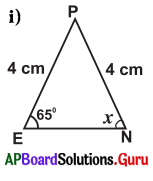Given, in ∆PEN, PE = 4 cm, PN = 4 cm ∠E = 65°, ∠N = x°
Two sides are equal in length.
So, ∆PEN is an isosceles triangle.

In isosceles triangle, the angles which are opposite to equal sides are also equal.
So, ∠N = ∠E
∴ x = 65°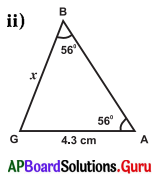Given, in ∆BGA,
∠B = 56°, ∠G = x, ∠A = 56° and BG = x cm, GA = 4.3 cm
We know that, sum of angles of a triangle is 180°.
In ∆BGA, ∠B + ∠G + ∠A = 180°
⇒ 56° + x + 56° = 180°
⇒ 112° + x = 180° :
⇒ 112° + x – 112° =180°- 112°
∴ x = 68°

Two angles are equal in ∆BGA.
So, ∆BGA is an isosceles triangle.
In isosceles triangle the sides which are opposite to equal angles are also equal.
So, BG = GA
∴ x = 4.3 cm

Question 4.
∆ABC is an isosceles triangle in which AB = AC. If ∠A = 80°, then find ∠B and ∠C.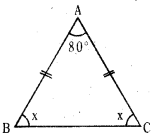In isosceles triangle the angles which are opposite to equal sides are also equal.
So, ∠B = ∠C = x°
In ∆ABC, we know
∠A + ∠B + ∠C — 180°
⇒ 80° + x + x = 180°
⇒ 80° + 2x – 80° = 180° – 80°
⇒ 2x = 100°
⇒ 2𝑥2=1002
∴ x = 50°
∴ ∠B = ∠C = 50°

Question 5.
Find the values of ‘x’ in each of the below triangles.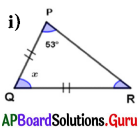In ∆PQR, PQ = QR and ∠P = 53°
∆PQR is an isosceles triangle.

In isosceles triangle, the angles which are opposite to equal sides are also equal.
So, ∠P = ∠R = 53°
In ∆PQR, we know
∠P + ∠Q + ∠R = 180°
⇒ 53° + x + 53° = 180°
⇒ 106° + x = 180°
⇒ 106° + x- 106° = 180°- 106°
∴ x = 74°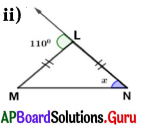In ∆LMN, LM = LN and exterior angle at L = 110° .
∆LMN is an isosceles triangle.
In isosceles triangle, the angles which are opposite to equal sides are also equal.

So, ∠M = ∠N = x° .
We know that an exterior angle of a triangle is equal to the sum of its opposite interior angles.
Exterior angle at
L = ∠M + ∠N = 110°
⇒ x + x = 110°
⇒ 2x = 110°
⇒ 2𝑥2=1102
∴ x = 55°

Question 6.
Which of the following statements are true in the following diagram.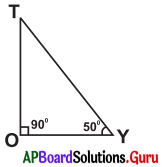(i) OY < OT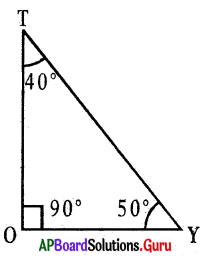In ∆TOY, ∠T + ∠O + ∠Y = 180°
⇒ ∠T + 90° + 50° = 180°
⇒ ∠T + 140° = 180°
⇒ ∠T + 140°- 140° = 180° – 140°
∴ ∠T – 40°
We know that side opposite to the largest angle is longest.
OY< OT – True

(ii) TY < TO
TY < TO False

(iii) ∠Y < ∠T
∠Y < ∠T
50° < 40° – False

(iv) TY < OY
TY < OY – False

## Andhra Pradesh Board Class 7th Maths Chapter 5 Triangles Ex 5.4 Textbooks for Exam Preparations

Andhra Pradesh Board Class 7th Maths Chapter 5 Triangles Ex 5.4 Textbook Solutions can be of great help in your Andhra Pradesh Board Class 7th Maths Chapter 5 Triangles Ex 5.4 exam preparation. The AP Board STD 7th Maths Chapter 5 Triangles Ex 5.4 Textbooks study material, used with the English medium textbooks, can help you complete the entire Class 7th Maths Chapter 5 Triangles Ex 5.4 Books State Board syllabus with maximum efficiency.

## FAQs Regarding Andhra Pradesh Board Class 7th Maths Chapter 5 Triangles Ex 5.4 Textbook Solutions

#### Can we get a Andhra Pradesh State Board Book PDF for all Classes?

Yes you can get Andhra Pradesh Board Text Book PDF for all classes using the links provided in the above article.

## Important Terms

Andhra Pradesh Board Class 7th Maths Chapter 5 Triangles Ex 5.4, AP Board Class 7th Maths Chapter 5 Triangles Ex 5.4 Textbooks, Andhra Pradesh State Board Class 7th Maths Chapter 5 Triangles Ex 5.4, Andhra Pradesh State Board Class 7th Maths Chapter 5 Triangles Ex 5.4 Textbook solutions, AP Board Class 7th Maths Chapter 5 Triangles Ex 5.4 Textbooks Solutions, Andhra Pradesh Board STD 7th Maths Chapter 5 Triangles Ex 5.4, AP Board STD 7th Maths Chapter 5 Triangles Ex 5.4 Textbooks, Andhra Pradesh State Board STD 7th Maths Chapter 5 Triangles Ex 5.4, Andhra Pradesh State Board STD 7th Maths Chapter 5 Triangles Ex 5.4 Textbook solutions, AP Board STD 7th Maths Chapter 5 Triangles Ex 5.4 Textbooks Solutions,
Share: